Courses

# Test: Friction Clutches - 3

## 10 Questions MCQ Test Topicwise Question Bank for Mechanical Engineering | Test: Friction Clutches - 3

Description
This mock test of Test: Friction Clutches - 3 for Mechanical Engineering helps you for every Mechanical Engineering entrance exam. This contains 10 Multiple Choice Questions for Mechanical Engineering Test: Friction Clutches - 3 (mcq) to study with solutions a complete question bank. The solved questions answers in this Test: Friction Clutches - 3 quiz give you a good mix of easy questions and tough questions. Mechanical Engineering students definitely take this Test: Friction Clutches - 3 exercise for a better result in the exam. You can find other Test: Friction Clutches - 3 extra questions, long questions & short questions for Mechanical Engineering on EduRev as well by searching above.
QUESTION: 1

### The frictional torque transmitted in a flat pivot bearing assuming uniform wear

Solution:

Frictional troque transmitted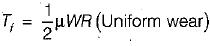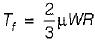(Uniform pressure)
where
μ = Coefficient of friction
W = Load over the bearing

QUESTION: 2

### A single clutch operating under dry sliding condition transmits 150 Nm. The average coefficient of friction is 0.3 and maximum operating pressure is 0.5 MPa. The ratio between the outside radius and inner radius is 1.73. Under uniform wear conditions, if the outside radius of the clutch is to be minimum, the inside radius will have to be

Solution: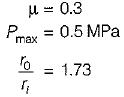Torque transmitted = 150 N-m
For uniform wear
p • r = Constant
0.5 x r1 = C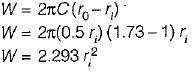Torque transmitted =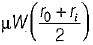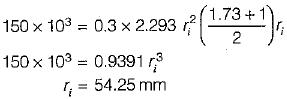QUESTION: 3

### Consider the following statements regarding a centrifugal clutch: 1. It need not be unloaded before engagement. 2. It enables the prime mover to start up under no-load conditions. 3. It picks up the load gradually with the increase in speed. 4. It will not slip to the point of destruction. 5. It is very useful when the power unit has a low starting torque. Q. Which of these are the advantages of a centrifugal clutch?

Solution:

Centrifugal clutch will have slip as it is example of friction clutch. it has to be unloaded before engagement.
Fc = mω2r

QUESTION: 4

A clutch has outer and inner diameters 100 mm and 40 mm respectively. Assuming a uniform pressure of 2 MPa and coefficient of friction of liner material 0.4, the torque carrying capacity of the clutch is

Solution:

Using uniform pressure theory, torque carrying capacity of clutch is given by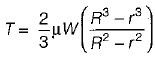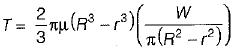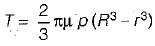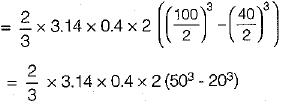= 195936 Nmm
= 195.936 Nm.

QUESTION: 5

A disc clutch is required to transmit 5 kW at 2000 rpm. The disc has a friction lining with coefficient of friction equal to 0.25. Bore radius of friction lining is equal 0.25 mm. Assume uniform contact pressure of 1 MPa. The value of outside radius of the friction lining is

Solution:

Power Transmitted = 5 kW
N = 2000 rpm
μ = 0.25
r1 = 25 mm
Uniform Pressure = 1 MPa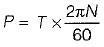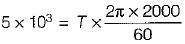T = 23.87 N-m
Pressure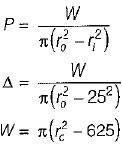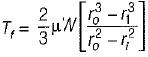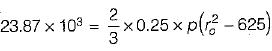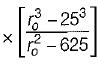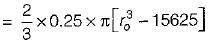ro= 39.4 mm
Bolts in a rigid flangecoupling fails in shear and crushing.

QUESTION: 6

Which one of the following statement is correct? In case of clutches, the uniform pressure theory:

Solution:
QUESTION: 7

Which one of the following is the correct expression for the torque transmitted by a conical clutch of outer radius R, inner radius r and semi-cone angle a assuming uniform pressure? (where W = total axial load and μ = coefficient of friction)

Solution:

Conical clutch, uniform pressure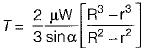where, α → semi angle of cone for uniform wear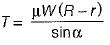QUESTION: 8

In a plate clutch axial force is 4 kN. The inside radius of contact surface is 50 mm and the outside radius is 100 mm. For uniform pressure the mean radius of friction surface will be

Solution: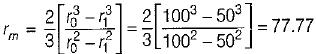QUESTION: 9

In the multiple disc clutch, if there are 6 discs on the driving shaft and 5 discs on the driven shaft, then the number of pairs of contact surfaces will be equal to

Solution:

n = n1 + n2 -1 = 6 + 5 - 1 = 10

QUESTION: 10

In cone clutch

Solution: